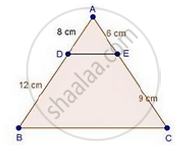Share

# D and E Are the Points on the Sides Ab and Ac Respectively of a δAbc Such That: Ad = 8 Cm, Db = 12 Cm, Ae = 6 Cm and Ce = 9 Cm. Prove that Bc = 5/2 De. - CBSE Class 10 - Mathematics

ConceptBasic Proportionality Theorem Or Thales Theorem

#### Question

D and E are the points on the sides AB and AC respectively of a ΔABC such that: AD = 8 cm, DB = 12 cm, AE = 6 cm and CE = 9 cm. Prove that BC = 5/2 DE.

#### SolutionWe have,

"AD"/"DB"=8/12=2/3

And, "AE"/"EC"=6/9=2/3

Since, "AD"/"DB"="AE"/"EC"

Then, by converse of basic proportionality theorem

DE || BC

∠A = ∠A                              [Common]

Then, ΔADE ~ ΔABC              [By AA similarity]

therefore"AD"/"AB"="DE"/"BC"                   [Corresponding parts of similar Δ are proportional]

rArr8/20="DE"/"BC"

rArr2/5="DE"/"BC"

"BC"=5/2" DE"

Is there an error in this question or solution?

#### Video TutorialsVIEW ALL 

Solution D and E Are the Points on the Sides Ab and Ac Respectively of a δAbc Such That: Ad = 8 Cm, Db = 12 Cm, Ae = 6 Cm and Ce = 9 Cm. Prove that Bc = 5/2 De. Concept: Basic Proportionality Theorem Or Thales Theorem.
S